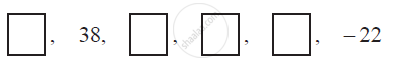Share

# In the following APs, find the missing terms in the boxes - CBSE Class 10 - Mathematics

#### Question

In the following APs, find the missing terms in the boxes :#### Solution

For this A.P.,

a2 = 38

a6 = −22

We know that

an = a + (n − 1) d

a2 = a + (2 − 1) d

38 = a + d (1)

a6 = a + (6 − 1) d

−22 = a + 5d (2)

On subtracting equation (1) from (2), we obtain

− 22 − 38 = 4d

−60 = 4d

d = −15

a = a2 − d = 38 − (−15) = 53

a3 = a + 2d = 53 + 2 (−15) = 23

a4 = a + 3d = 53 + 3 (−15) = 8

a5 = a + 4d = 53 + 4 (−15) = −7

Therefore, the missing terms are 53, 23, 8, and −7 respectively.

Is there an error in this question or solution?

#### APPEARS IN

NCERT Solution for Mathematics Textbook for Class 10 (2019 to Current)
Chapter 5: Arithmetic Progressions
Ex. 5.20 | Q: 3.5 | Page no. 106
Solution In the following APs, find the missing terms in the boxes Concept: nth Term of an AP.
S